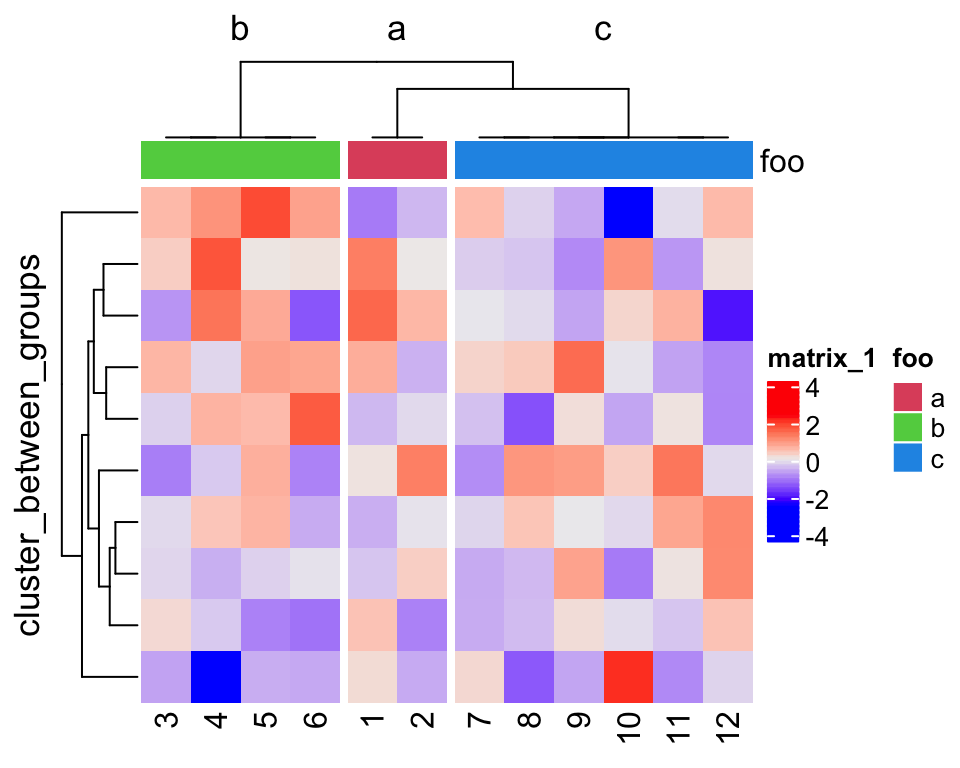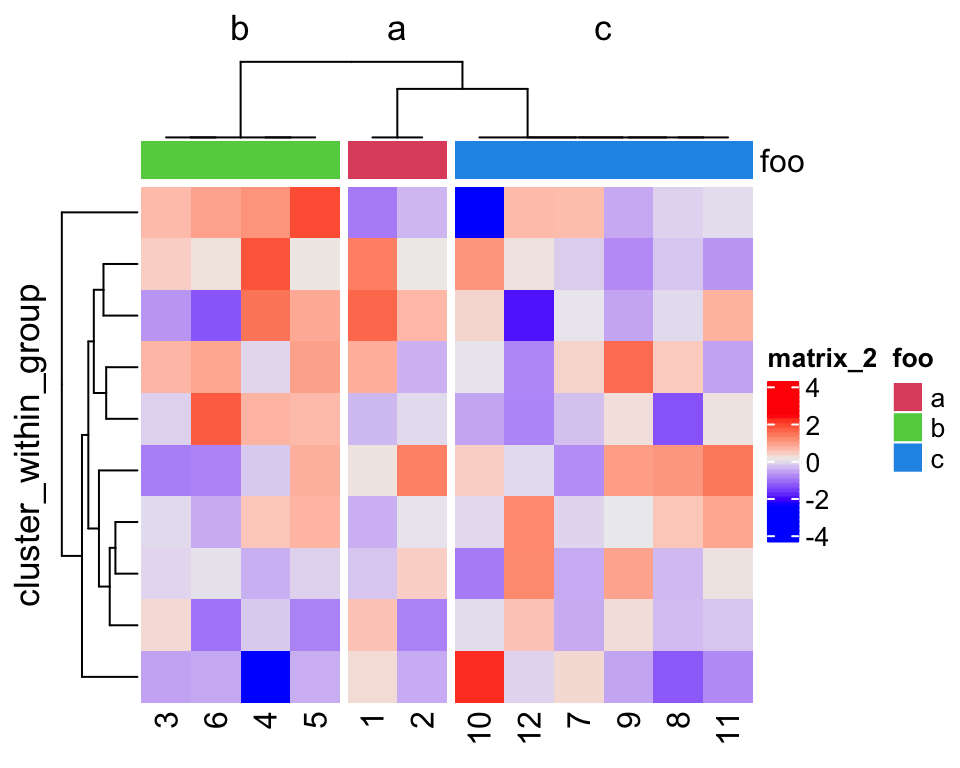# Cluster groups in ComplexHeatmap

When making heatmaps, we always have many additionally grouping information for the samples, sush as subtypes, phenotypes. To emphasize the difference between groups, we might want to only perform clustering on group level, while not on the complete dataset. For these scenarios, ComplexHeatmap has two functions: `cluster_between_groups()` and `cluster_within_group()`. The two functions all perform clustering on group level. The difference is, in `cluster_between_groups()`, inside each group, the order of samples is unchanged, while in `cluster_within_group()`, samples in each group are still clustered.

I demonstrate the use of the two functions with a simple random matrix. In the first plot, `cluster_between_groups()` was applied. The column names correspond to the original order in the matrix, so you can observe whether the columns are reordered or not.

``````library(ComplexHeatmap)
set.seed(372)
m = matrix(rnorm(120), nc = 12)
colnames(m) = 1:12
fa = rep(c("a", "b", "c"), times = c(2, 4, 6))
fa_col = c("a" = 2, "b" = 3, "c" = 4)
dend1 = cluster_between_groups(m, fa)
Heatmap(m, cluster_columns = dend1, column_split = 3,
row_title = "cluster_between_groups",
top_annotation = HeatmapAnnotation(foo = fa, col = list(foo = fa_col)))``````In the second plot, `cluster_within_group()` was applied. As you can see, columns are also reordered inside each group.

``````dend2 = cluster_within_group(m, fa)
Heatmap(m, cluster_columns = dend2, column_split = 3,
row_title = "cluster_within_group",
top_annotation = HeatmapAnnotation(foo = fa, col = list(foo = fa_col)))``````Note, they also work on heatmap rows. Please update to the current GitHub version to use these two functions.

Finally, thanks @crazyhottommy for providing this interesting use case.